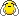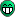# Number of entries in a tree

I have a tree which I’m analysing using MakeClass. I’ve modified the .C file so it reads a branch, event by event, and fills out a histogram. Judging by the number of entries in that histogram, it seems that I’m doing something wrong. The histogram has 1.24e07 entries. What confuses me is that when I plot the same branch in TBrowser, the number of entries in that histogram is 63287. Can someone explain me why is that so?
The file I’m using, together with .C and .h files, is here.

If TBrowser uses TTree::Draw under the hood, you might be running into the default “nentries” argument. Or there might be a setting for a max number of entries drawn via the browser.

I also notice you’re using GetEntriesFast() instead of GetEntries(). I don’t remember the difference, but try changing it to GetEntries() and see what happens.

Hi,

The issue is that your data is actually a collection (per TTree entry) that contains 0 or more elements. TTree::MakeClass emulated the collection with C style array and declared the variable you are using as:

``` Float_t StrSimHits_m_Edep[kMaxStrSimHits]; //[StrSimHits_] ```whose effective size is given by StrSimHits_. So in Loop you meant to write something like:

``````   for(unsigned int i = 0; i < StrSimHits_; ++i) {
if(StrSimHits_m_Edep[i] > 0) {
hedep -> Fill( StrSimHits_m_Edep[i] );
}
}``````

Cheers,
Philippe.

Thanks, that solved my problem!One more question:

If the declaration is:

How do I access the individual entries? Namely, I want

```for (unsigned int i = 0; i < StrSimHits_; ++i){ if(StrSimHits_m_Edep[i] > 0){ if(StrSimHits_m_Position.Z() < 0){ hedep -> Fill(StrSimHits_m_Edep[i]); } } }```
but this returns an empty histogram.

Thanks,

Andrea

StrSimHits_m_Position[i].Z()

Thanks, I no longer get an error, but my histogram is still empty and I know for sure it shouldn’t be. When I open the tree in browser, I see that the StrSimHits_m_Position branch has many leaves and most of them are empty except fX, fY, fZ. The empty ones are x(), y(), z(), Mag(), theta()…
If I do StrSimHits_m_Position[i].Z(), is it using the empty z(), or the fZ which I want to use?

It seems that the “MakeClass” generated analysis skeleton has problems with two branches of the “selectedTree” (all TVector3 seem to be 0, possibly not retrieved at all):

*Br 9 :StrSimHits.m_Momentum : TVector3 m_Momentum[StrSimHits_] *
*Br 10 :StrSimHits.m_Position : TVector3 m_Position[StrSimHits_] *

I guess Philippe would need to comment on it.

Hi,

The MakeClass skeleton does not properly handle class that can not be ‘split’ in their internal components.

To read the TVector3 part, you will need to either use the original object/class (by using either the original library or one generated by MakeProject) instead of the MakeClass technique or you could switch to using MakeSelector from version 6 (which using the TTreeReader rather than MakeClass).

Cheers,
Philippe.

Thanks, Phillipe!
I made a selector, but the positions are still not accurate. I am attaching the .C and .h files.
When I write out the z position, the values are ~6.94922e-310, when they should be between -150 and 250.
Am I doing something wrong?
StrSelector.h (3.39 KB)
StrSelector.C (4.81 KB)

If you try (ROOT 6.06/06):

``````std::cout << " " << StrSimHits_m_Edep.GetSize()
<< " " << StrSimHits_m_Momentum.GetSize()
<< " " << StrSimHits_m_Position.GetSize()
<< std::endl;
``````

you will see that both TVector3 related arrays are always empty (size = 0).

I see. Any suggestions on how to fix it?

Start to pray that Philippe knows.Actually not quite, there is 0.1% of entries that are not emptyHowever the real problem is still there … the TTreeReader indeed is using the wrong offset for the TVector3 (I think there actually already a related JIRA ticket) … I am investigating why this offset is wrong.

Cheers,
Philippe.

If I try something like this:

``````Bool_t StrSelector::Process(Long64_t entry) {
if ((StrSimHits_m_Momentum.GetSize() != 0) ||
(StrSimHits_m_Position.GetSize() != 0)) {
std::cout << " " << entry
<< " " << StrSimHits_m_Edep.GetSize()
<< " " << StrSimHits_m_Momentum.GetSize()
<< " " << StrSimHits_m_Position.GetSize()
<< std::endl;
}
return kTRUE;
}
``````

then I get no “verbose” output at all (ROOT 6.06/06).
Do you say that you get 0.1% of entries with non-empty TTreeReaderArray arrays?
Note: It is, of course, clear that the corresponding file-resident branches are NOT completely empty (so, I think it’s just the TTreeReader which fails to retrieve them).

BTW. Do you have any solution that Andrea (and others possibly) could use with any current version of ROOT (e.g 5.34/36 or 6.06/06)? Even if you fix this problem in the master, it’s really hard to expect that one will build the current master just to be able to analyse some old file.

A likely solution would be to use MakeProject in conjunction with (the result of) MakeProxy. This would give access to a full C++ version of the original objects.

I am saying that there is that many. To be exact:

``````root  tree->GetEntries("StrSimHits@.size()!=0")
(Long64_t) 19397
root  tree->GetEntries()
(Long64_t) 12402250``````

In addition, I see at least one with the TTreeReader, with code like:

``` StrSimHits_m_Edep.GetSize(); std::cout << " " << StrSimHits_m_Edep.GetSize() << " " << StrSimHits_m_Momentum.GetSize() << " " << StrSimHits_m_Position.GetSize() << " " << StrSimHits_m_CellId.GetSize() << std::endl; for(int i = 0, n = StrSimHits_m_Edep.GetSize(); i < n; ++i){ cout << "Z position " << StrSimHits_m_Position[i].z() << endl; if (StrSimHits_m_Position[i].Z() >=0){ ```

I get:

``` root  tree->Process(&s,"",3,38) 2 0 0 2 Z position 2.36446e-314 Z position 2.36446e-314 ```Where the Z position print are indeed wrong.

Cheers,
Philippe.

I assume that if “StrSimHits_m_Position.GetSize()” returns 0 and you try to access “StrSimHits_m_Position[i]” then you are actually reading some random RAM contents (hence the strange 2.36446e-314 value).

I also noticed that TTree::Draw and TTree::Project seem to deliver reasonable results (that’s actually how I discovered that the file-resident branches were not empty).
Maybe that’s the simplest solution for the time being, e.g. something like this seems to work with ROOT 5.34 and 6.06:

``````{
TH1::SetDefaultSumw2(kTRUE);
TFile *fin = TFile::Open("output.root");
TTree *t; fin->GetObject("selectedTree", t);
TCanvas *c = new TCanvas("c", "c");
c->Divide(2, 2);
c->cd(1);
t->Draw("StrSimHits.m_Position.Z()", "", "HIST E");
c->cd(2);
t->Draw("StrSimHits.m_Edep", "StrSimHits.m_Position.Z() >= 0", "HIST E");
c->cd(3);
t->Draw("1*(StrSimHits.m_Position.Z() >= 0) + 3*(StrSimHits.m_Position.Z() < 0)", "StrSimHits.m_Edep", "HIST E");
c->cd(4);
TH1F *htedep = new TH1F("htedep", "total Edep per crystal", 8, 0, 8);
t->Project("htedep", "1*(StrSimHits.m_Position.Z() >= 0) + 3*(StrSimHits.m_Position.Z() < 0)", "StrSimHits.m_Edep");
htedep->Draw("HIST E");
c->cd(0);
TFile *fout = TFile::Open("results.root", "RECREATE");
htedep->Write("Edep");
delete fout;
htedep->SetDirectory(gROOT); // "disconnect" from "fin"
delete fin; // automatically deletes "t", too
// delete htedep; // when you no longer need it
// delete c; // when you no longer need it
}
``````

[quote]I assume that if “StrSimHits_m_Position.GetSize()” returns 0 and you try to access “StrSimHits_m_Position[i]” then you are actually reading some random RAM contents (hence the strange 2.36446e-314 value).[/quote]Unfortunately, not that simple. The GetSize is not zero as the printout are within a loop based on the size … the problem is really that TTreeReader is using the wrong offset within the object …

Thanks,
Philippe.

Thanks both, I will try your suggestions and see how it goes.

I was talking to my colleague and he says he is usually using MakeSelector to analyze trees with TVector3’s, so I’m curious, why is this tree different than any other and MakeSelector is not working?